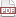### Tip 53: Count references

15 January 12. [link]PDF version

level: your data structures are getting busy
purpose: Know when to hold em; fold em

Part of a series of tips on POSIX and C. Start from the tip intro page, or get 21st Century C, the book based on this series.

Last time, we saw the situation where there was one data set being viewed many times, meaning that one struct was canonical and the others, although otherwise identical, were subsidiary.

This time, we'll point to the same data many times over, and each pointer will be identical. After we have two or three references to the data, we won't know or care which came first. But the problem is similar: we need to free the struct and its contents when there are no references to it left, and no sooner.

Adding an owner bit to the structure was an easy four-step process last time, and it'll be approximately the same process this time:

• The type definition includes an integer named counter.
• The new function sets counter = 1.
• The boilerplate copy function sets counter++.
• The free function queries if(-counter==0), and if yes, then free all shared data; else, just leave everything as is, because we know there are still references to the structure.

Again, as long as your work with the structure is entirely via the new/copy/free functions, this will work fine.

Here's another full example. It is an agent-based model of group membership. Agents are on a two-dimensional preference space (because we'll plot the groups) in the square between (- 1, - 1) and (1, 1). Their utility from a group is -(distance to group's mean position + M*number of members). The group's mean position is simply the mean of the positions of the group's members (excluding the agent querying the group), and M is a constant that scales how much the agents care about being in a large group relative to how much they care about the group's mean position. At each round, agents will join the group with the best utility to the agent.

With some random odds, the agent will originate a new group. However, because agents are picking a new group every period, the agent may abandon that newly originated group in the next period.

Brace yourself--this takes almost 125 lines of code.

The header. What I call the join and exit functions might more commonly be read as the copy and free functions. The group_t structure has a size element, which is the number of group members--the reference count. You can see that I use Apophenia and Glib. Notably, the groups are held in a linked list, private to the groups.c file; maintaining that list will require fully two lines of code, including a call to g_list_append and g_list_remove.

#include <apop.h>
#include <glib.h>

typedef struct {
gsl_vector *positions;
int id, size;
} group_t;

group_t* group_new(gsl_vector *positions);
group_t* group_join(group_t *joinme, gsl_vector *position);
void group_exit(group_t *leaveme, gsl_vector *position);
group_t* group_closest(gsl_vector *position, double mb);
void print_groups();


Now for the file defining the details of the group object. Notice that the printout function is Gnuplot friendly. If you use another plotting system, it shouldn't take but a few seconds to rewrite it as needed.

#include "groups.h"

GList *group_list;

group_t *group_new(gsl_vector *positions){
static int id=0;
group_t *out = malloc(sizeof(group_t));
*out = (group_t) {.positions=apop_vector_copy(positions), .id=id++, .size=1};
group_list = g_list_append(group_list, out);
return out;
}

//The copy function
group_t *group_join(group_t *joinme, gsl_vector *position){
int n = ++joinme->size;  //increment the reference count
for (int i=0; i< joinme->positions->size; i++){
joinme->positions->data[i] *= (n-1.)/n;
joinme->positions->data[i] += position->data[i]/n;
}
return joinme;
}

//The free function
void group_exit(group_t *leaveme, gsl_vector *position){
int n = leaveme->size--;  //lower the reference count
for (int i=0; i< leaveme->positions->size; i++){
leaveme->positions->data[i] -= position->data[i]/n;
leaveme->positions->data[i] *= n/(n-1.);
}
if (leaveme->size == 0){ //garbage collect?
gsl_vector_free(leaveme->positions);
group_list= g_list_remove(group_list, leaveme);
free(leaveme);
}
}

group_t *group_closest(gsl_vector *position, double mass_benefit){
group_t *fave=NULL;
double smallest_dist=GSL_POSINF;
for (GList *gl=group_list; gl!= NULL; gl = gl->next){
group_t *g = gl->data;
double dist= apop_vector_distance(g->positions, position, 'L', 3)-
mass_benefit*g->size;
if(dist < smallest_dist){
smallest_dist = dist;
fave = g;
}
}
return fave;
}

void print_groups(){
printf("plot '-' with points pointtype 6\n");
for (GList *gl=group_list; gl!= NULL; gl = gl->next)
apop_vector_print(((group_t*)gl->data)->positions);
printf("e\n");
}


The program file, defining the array of persons, and the main loop of rechecking memberships and printing out. At this point all interface with the groups happens via the new/join/exit/print functions. There is zero memory management code in this file--the reference counting guarantees us that when the last member exits the group, it will be freed.

Again, main does some Gnuplot-specific stuff, so if you saved this as groupabm.c, then call groupabm | gnuplot on your command line. You can watch the group centers space out, and occasionally merge or split.

#include "groups.h"

int pop=2000,
periods=200,
dimension=2;

typedef struct {
gsl_vector *positions;
group_t *group;
} person_t;

void check_membership(person_t *p, gsl_rng *r, double mass_benefit, double new_group_odds){
group_exit(p->group, p->positions);
p->group=NULL;
if (!p->group) p->group =
(gsl_rng_uniform(r) < new_group_odds)
? group_new(p->positions)
: group_join(group_closest(p->positions, mass_benefit), p->positions);
}

person_t person_setup(gsl_rng *r){
gsl_vector *posn = gsl_vector_alloc(dimension);
for (int i=0; i< dimension; i++)
gsl_vector_set(posn, i, 2*gsl_rng_uniform(r)-1);
return (person_t){.positions=posn};
}

void init(person_t *people, int pop, gsl_rng *r){
for (int i=0; i< pop; i++)
people[i] = person_setup(r);
for (int i=0; i< 10; i++)
people[i].group = group_new(people[i].positions);
for (int i=10; i< pop; i++)
people[i].group = group_join(people[i%10].group, people[i].positions);
}

int main(){
double  new_group_odds = 1./pop,
mass_benefit = .7/pop;
gsl_rng *r = apop_rng_alloc(1234);
person_t people[pop];
init(people, pop, r);
printf("unset key;set xrange [-1:1]\nset yrange [-1:1]\n");
for (int t=0; t< periods; t++){
print_groups();
for (int i=0; i< pop; i++)
check_membership(&people[i], r, mass_benefit, new_group_odds);
}
}